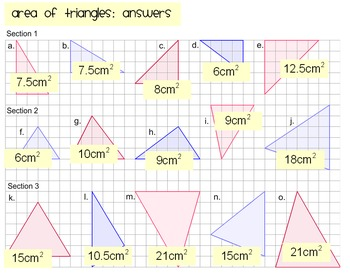# Area of TrianglesSubject
Resource Type
File Type
Zip (3 MB|10 pages)
Standards
\$2.50
• Product Description
• Standards
Area of Triangles : Lesson Plan & Activity Pack

This pack contains activities and prompts for a lesson to help students understand the formula for the area of a triangle.

It contains:
1. Area of a Triangle Powerpoint Presentation & Lesson Plan
Lots of diagrams to discuss with students to help them understand where the formula comes from.

2. Area of a Triangle Introductory worksheet
This worksheet gradually increases in difficulty to encourage students to think of quick ways to find the area of a triangle.

3. Area of a Triangle: Match Three!
Students work out the area of triangles and match them into groups of three triangles with the same area.

4. Area of Triangles: Extra Practise.
This could be used for a bit of extra help, or could be set as a homework task.

5. Biggest Area Worksheet
When students have mastered the basics, this activity requires them to measure a set of triangles drawn on plain paper in order to work out which triangle in each row is biggest.

\$\$ Save \$\$
Buy this as part of my area and volume bundle and save \$\$!

Tags: Area of Triangles, area, measurement, area of right triangles.

Common Core: 6.G.A.1
Find the area of right triangles, other triangles, special quadrilaterals, and polygons by composing into rectangles or decomposing into triangles and other shapes; apply these techniques in the context of solving real-world and mathematical problems.
Total Pages
10 pages